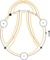# Applied Mathematics

Applied mathematics is a branch of mathematics that deals with mathematical methods that find use in science, engineering, business, computer science, and industry. Thus, applied mathematics is a combination of mathematical science and specialized knowledge. The term "applied mathematics" also describes the professional specialty in which mathematicians work on practical problems by formulating and studying mathematical models. In the past, practical applications have motivated the development of mathematical theories, which then became the subject of study in pure mathematics where abstract c ...more

## Applied Mathematics BooksElectromagnetic Intera...

## Groups Tagged "Applied Mathematics"This group is for people interested in mathematics at the college level. All are welcome. Profes…more
555 members, last active one year ago